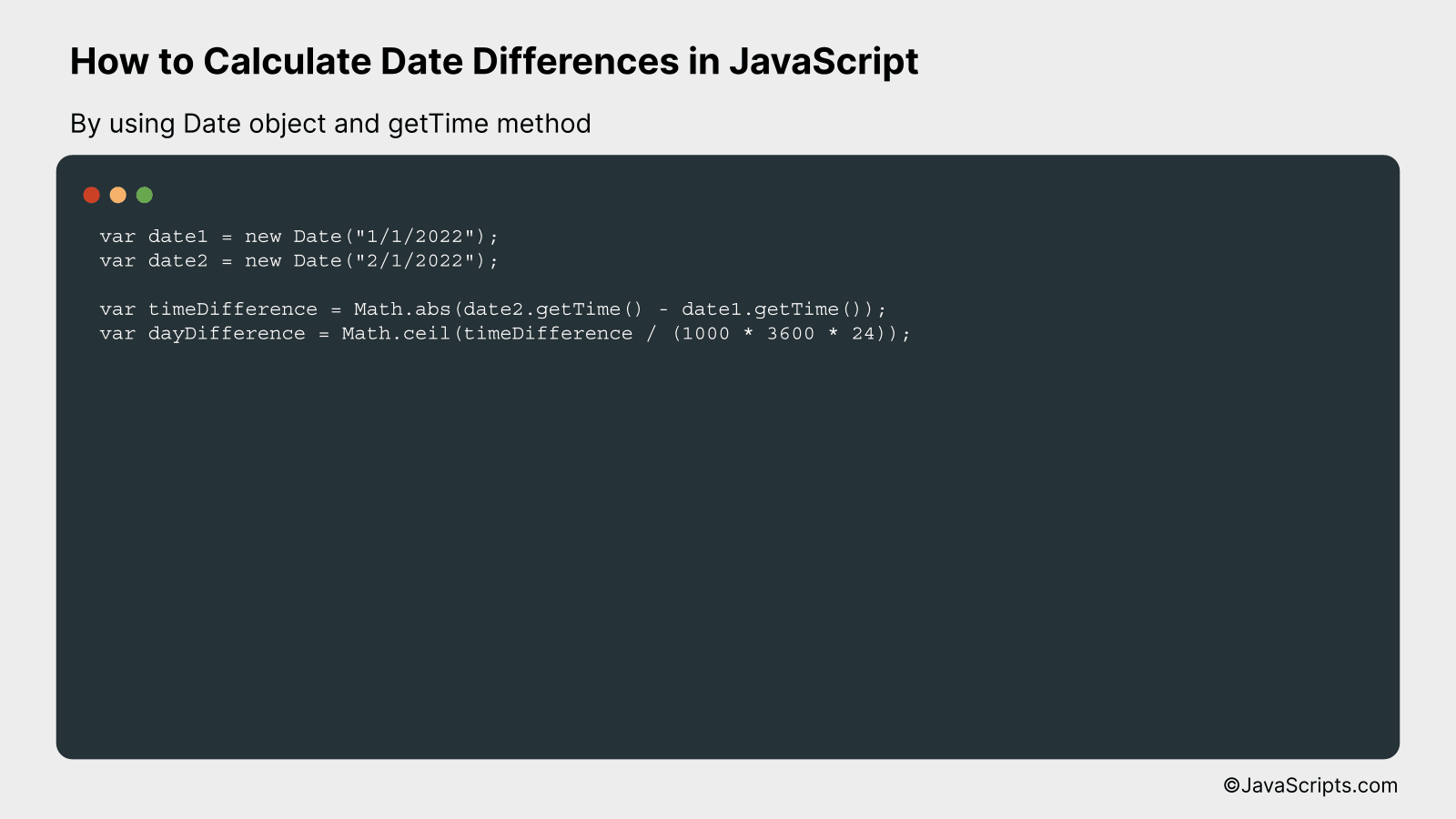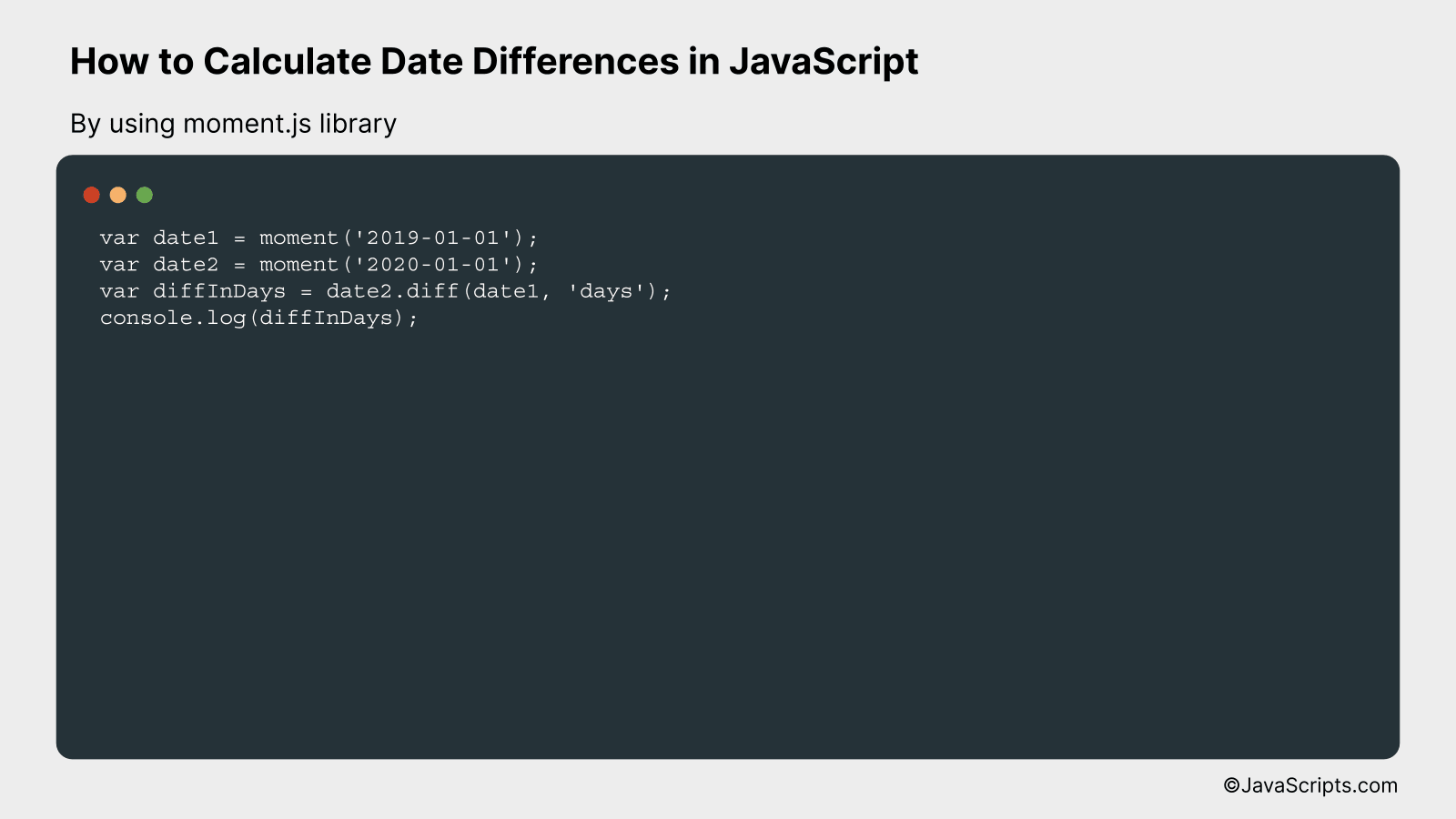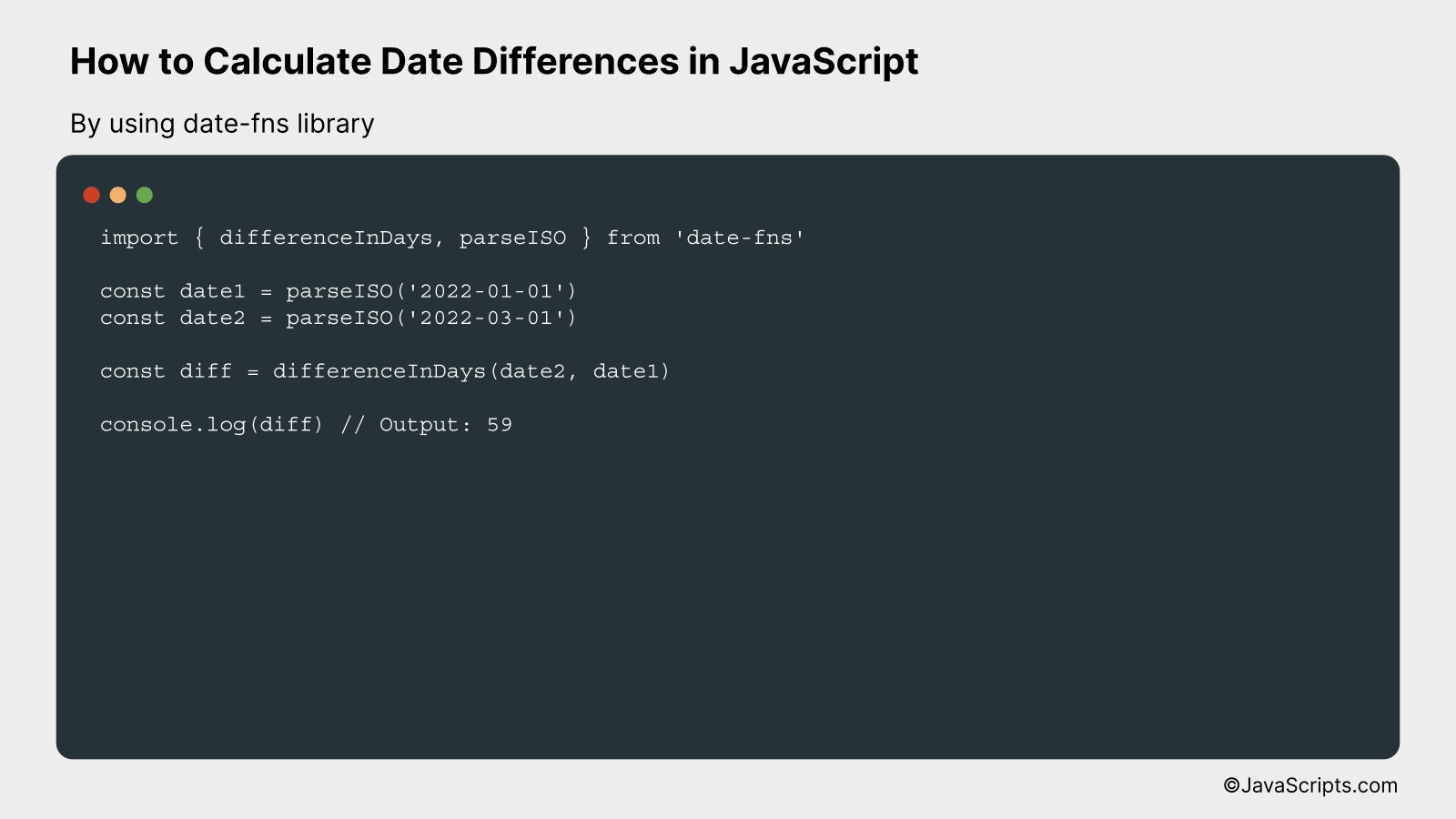# How to Calculate Date Differences in JavaScript

Do you ever find yourself needing to calculate the difference between two dates when programming in JavaScript? It’s a common requirement, especially when working with event scheduling apps, calendars, or even project management systems.

Let’s dive into how JavaScript can make this task simpler for you. Together, we’ll explore various methods to calculate date differences, ensuring you have the necessary tools to handle any date-related calculations in your projects.

In this post, we will be looking at the following 3 ways to calculate date differences in JavaScript:

• By using Date object and getTime method
• By using moment.js library
• By using date-fns library

Let’s explore each…

## #1 – By using Date object and getTime methodWe will calculate date differences in JavaScript by subtracting two Date objects, which gives the difference in milliseconds, then converting that to the desired units (seconds, minutes, hours, days, etc.). For instance, if you want to find out how many days are between January 1, 2022 and February 1, 2022, you can use this method.

``````
var date1 = new Date("1/1/2022");
var date2 = new Date("2/1/2022");

var timeDifference = Math.abs(date2.getTime() - date1.getTime());
var dayDifference = Math.ceil(timeDifference / (1000 * 3600 * 24));
``````

### How it works

Each Date object represents a specific point in time, and the getTime method returns the numeric value corresponding to the time for the specified date according to universal time, which is the number of milliseconds since January 1, 1970, 00:00:00 UTC. By subtracting the getTime value of one date from another, we get the difference in milliseconds, which we can then convert into any unit of time we desire.

• The Date objects are created using the “new Date()” constructor and the specific dates are passed as strings in the format “mm/dd/yyyy”.
• The getTime method is used to get the time in milliseconds for each date from January 1, 1970.
• The absolute value of the difference between these two times in milliseconds is calculated using Math.abs to ensure the result is always positive, regardless of the order of the dates.
• The time difference in milliseconds is then converted into days by dividing by the number of milliseconds in a day (1000 milliseconds * 3600 seconds * 24 hours).
• Finally, Math.ceil is used to round up to the nearest whole number, to provide the total number of full days between the two dates.

## #2 – By using moment.js libraryWe are going to calculate the difference between two dates in JavaScript using the moment.js library. For example, if we have two dates – ‘2019-01-01’ and ‘2020-01-01’, we can calculate the exact difference in days by using moment’s diff function.

``````
var date1 = moment('2019-01-01');
var date2 = moment('2020-01-01');
var diffInDays = date2.diff(date1, 'days');
console.log(diffInDays);
``````

### How it works

The moment.js library is used to handle dates in JavaScript. The ‘diff’ function of moment.js calculates the difference between two dates. In the above code, we are calculating the difference in ‘days’ but it can be used to calculate difference in ‘years’, ‘months’, ‘weeks’, ‘hours’, ‘minutes’, and ‘seconds’ as well.

• Step 1: We create two moment objects by passing the dates in ‘YYYY-MM-DD’ format. ‘date1’ is ‘2019-01-01’ and ‘date2’ is ‘2020-01-01’.
• Step 2: We use the ‘diff’ function of moment.js which takes two arguments. The first argument is the date from which we want to calculate the difference, and the second argument is the unit of time for the difference. In our case, the unit of time is ‘days’.
• Step 3: The ‘diff’ function returns the difference in the unit of time specified. We store this result in the ‘diffInDays’ variable.
• Step 4: We log the difference to the console using ‘console.log’.

## #3 – By using date-fns libraryUsing the date-fns JavaScript library, we can easily calculate the difference between two dates by subtracting them and then formatting the result to display the difference in days. Let’s take an example where we calculate the number of days between January 1, 2022 and March 1, 2022.

``````
import { differenceInDays, parseISO } from 'date-fns'

const date1 = parseISO('2022-01-01')
const date2 = parseISO('2022-03-01')

const diff = differenceInDays(date2, date1)

console.log(diff) // Output: 59
``````

### How it works

This script calculates the difference in days between two dates by leveraging the ‘differenceInDays’ and ‘parseISO’ functions from the date-fns library. The ‘parseISO’ is used to convert a string in ISO 8601 format to a date object, and the ‘differenceInDays’ function calculates the difference between the two dates.

• First, we import the necessary functions from the date-fns library: ‘differenceInDays’ and ‘parseISO’.
• Next, we use ‘parseISO’ to create two date objects, ‘date1’ and ‘date2’, from strings in ISO 8601 format (‘2022-01-01’ and ‘2022-03-01’).
• We then use the ‘differenceInDays’ function to calculate the difference in days between ‘date2’ and ‘date1’. This will return a positive integer if ‘date2’ is after ‘date1’, a negative integer if ‘date1’ is after ‘date2’, and 0 if the dates are the same.
• Finally, we log the result to the console. In this case, the output will be 59, as there are 59 days between January 1, 2022 and March 1, 2022.

Related:

In conclusion, calculating date differences in JavaScript is an essential skill for any web developer. Whether you’re developing an event countdown, a project deadline tracker, or a data analytics tool, understanding and implementing date differences can empower your applications immensely.

It’s all about extracting the required information from Date objects and performing basic arithmetic operations. Remember, while JavaScript provides a range of built-in methods, external libraries like Moment.js can simplify the process further. Keep practicing, and you’ll master this in no time.